Courses

# Test: Governors - 3

## 10 Questions MCQ Test Theory of Machines (TOM) | Test: Governors - 3

Description
This mock test of Test: Governors - 3 for Mechanical Engineering helps you for every Mechanical Engineering entrance exam. This contains 10 Multiple Choice Questions for Mechanical Engineering Test: Governors - 3 (mcq) to study with solutions a complete question bank. The solved questions answers in this Test: Governors - 3 quiz give you a good mix of easy questions and tough questions. Mechanical Engineering students definitely take this Test: Governors - 3 exercise for a better result in the exam. You can find other Test: Governors - 3 extra questions, long questions & short questions for Mechanical Engineering on EduRev as well by searching above.
QUESTION: 1

### For a given fractional change of speed. If the displacement of the sleeve is high, then the governor is said to be

Solution:

A Governor is said to be sensitive when it readily responds to a small change of speed. The movement of the sleeve for a fractional change of speed is measure of sensitivity.

QUESTION: 2

### Effect of friction, at the sleeve of a centrifugal governor is to make it

Solution:

Friction at the sleeve makes the governor less sensitive.

QUESTION: 3

### The term “effort of governor” refers to

Solution:

The effort of a governor is the mean force exerted at the sleeve for a given percentage change of speed.

QUESTION: 4

Which one of the following statements is correct? A governor will be stable if the radius of rotation of the balls

Solution:

For stable governor
F = Ar - B
and F = mrω2
∴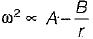QUESTION: 5

For a governor running at constant speed, what is the value of the force acting on the sleeve

Solution:

When governor is running at constant speed, then the net force acting of sleeve is zero.

QUESTION: 6

The problem of hunting of a centrifugal governor becomes very acute when the governor becomes

Solution:

Sensitiveness of a governor is a desirable quality. However, if a governor is too sensitive, it may fluctuate continuously above and below the mean position, it is called hunting of governor.

QUESTION: 7

The nature of the governors is shown by the graph between radius (r) of rotation and controlling force (f). Which of the following is an isochronous governor?.

Solution:

For isochronous governor
f = ar
Hence plot will be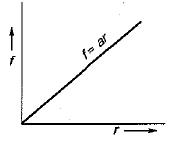QUESTION: 8

In a governor, the controlling force curve shows a relationship between

Solution:

Controlling force curve is plotted between controlling force at y-axis and radius of rotation at x-axis.

QUESTION: 9

The angular speed of a watt’s governor when its height is 20 cm, will be equal to

Solution: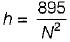where, h = height of governor
N = RPM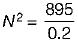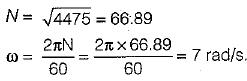QUESTION: 10

In a spring-controlled governor the controlling force curve is straight line. The balls are 400 mm apart when the controlling force is 1600 N and they are 240 mm apart when the force is 800 N. To make the governor isochronous the initial tension must be increased by

Solution:

The controlling force curve of a spring controlled governor is straight line and thus can be expressed as
f = ar+ b
when f = 1600 N, r = 400 mm
when f = 800 N, r = 240 mm
1600 = a(0.4) + b ...(i)
800 = a(0.24) + b ...(ii)
By solving (i) and (ii) we get
a = 5000 N/m
b = -400 N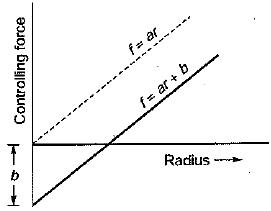To make the governor isochronous, the controlling force line must pass through the origin. This is possible only if the initial tension is increased by 400 N.